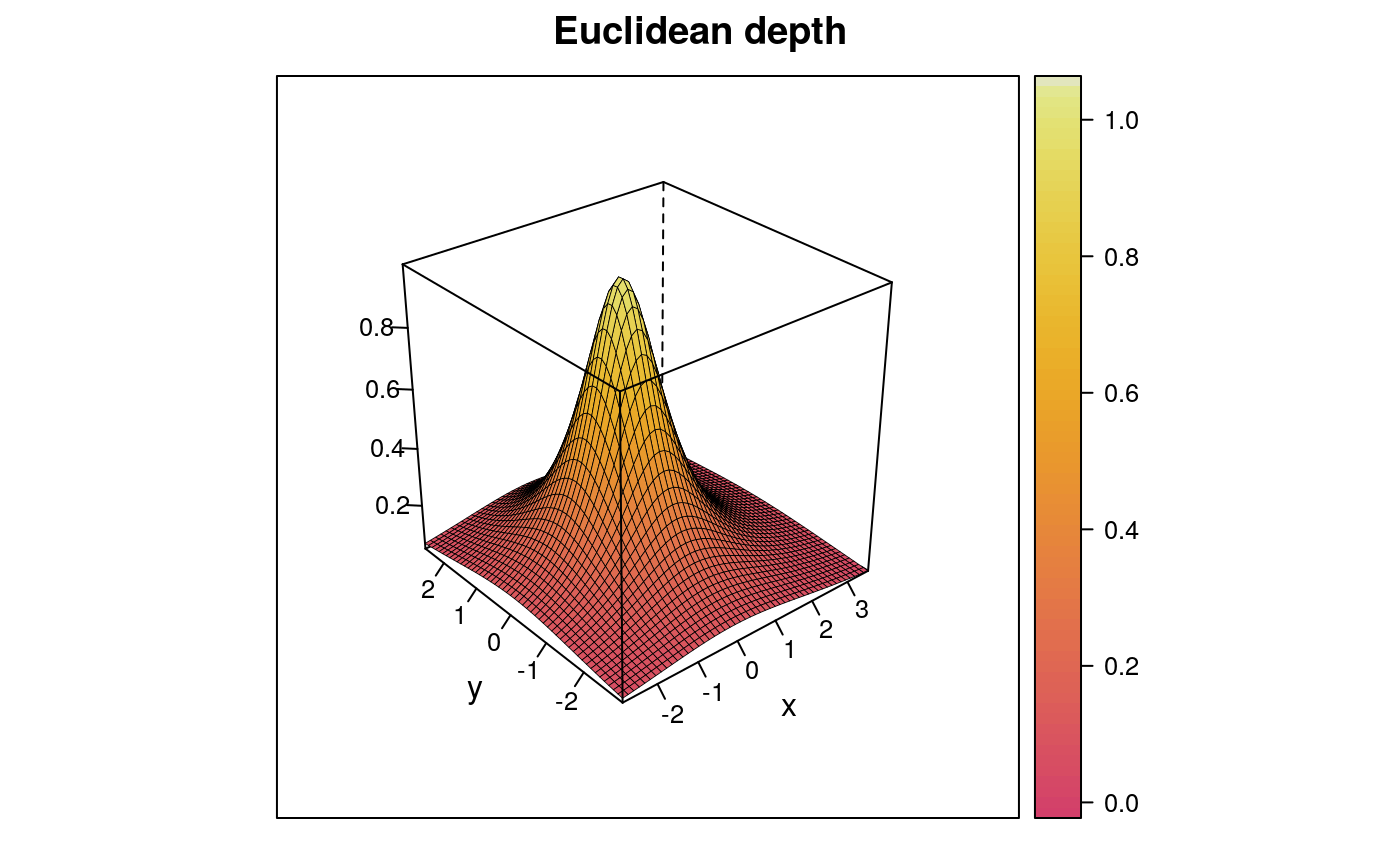Draws a perspective plot of depth function over x-y plane.

depthPersp(
x,
plot_method = "lattice",
xlim = extendrange(x[, 1], f = 0.1),
ylim = extendrange(x[, 2], f = 0.1),
n = 50,
xlab = "x",
ylab = "y",
plot_title = NULL,
colors = heat_hcl,
depth_params = list(),
graph_params = list()
)

## Arguments

x bivariate data there are two options "lattice", and "rgl" --- see details limits for x-axis limits for y-axis number of points that will be used to create plot ($$n ^ 2$$) description of x-axis description of y-axis plot title (default NULL means paste(depth_params\$method, "depth")) function for colors pallete (e.g. gray.colors). list of parameters for function depth ("method", "threads", "ndir", "la", "lb", "pdim", "mean", "cov", "exact"). list of graphical parameters for functions rgl::persp3d and lattice::wireframe.

## Details

plot_method --- rgl package is not in depends list beacuse it may cause problems when OpenGL is not supported. To use plot_method = "rgl" you must load this package on your own.

## Examples

# EXAMPLE 1
x <- mvrnorm(100, c(0, 0), diag(2))
depthPersp(x, depth_params = list(method = "Euclidean"))# EXAMPLE 2
data(inf.mort, maesles.imm)
data1990 <- na.omit(cbind(inf.mort[, 1], maesles.imm[, 1]))

if (FALSE) {
library(rgl)
depthPersp(data1990, plot_method = "rgl",
depth_params = list(method = "Projection"))
}